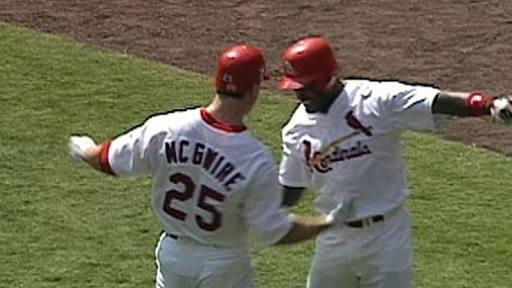# What Do You Know About Maris–mcgwire–sosa Pair?

10 Questions | Total Attempts: 103SettingsFamously inspired by baseball players Roger Maris, Mark McGwire, and Sammy Sosa, Maris–McGwire–Sosa pairs (or MMG pairs/numbers) are used in recreational mathematics to denote a particular situation concerning two consecutive natural numbers. The concept was named so by Mike Keith, an engineer and mathematician from the United States.

• 1.
Which pairs are very similar to Maris–McGwire–Sosa pairs?
• A.

Ruth–Aaron pairs

• B.

Bulgarian numbers

• C.

Monastery-church pairs

• D.

Factor pairs

• 2.
What is the birth name of the mathematician who named the puzzle?
• A.

Mike Keith

• B.

Mikel Keith

• C.

Micheal Keith

• D.

Michael Keith

• 3.
How many consecutive natural numbers are used in the puzzle?
• A.

2

• B.

4

• C.

6

• D.

8

• 4.
The addition of a number's digit—in base 10—to the digits of its prime factorization gives rise to which of the following?
• A.

The same sum

• B.

A different value

• C.

The number greater than 10

• D.

The number less than 10

• 5.
What is the total number of home runs attained by Mark McGwire and Sammy Sosa?
• A.

59

• B.

60

• C.

61

• D.

62

• 6.
Are 61 and 62 a Maris–McGwire–Sosa pair?
• A.

Yes

• B.

No

• C.

Yes, if and only if an integer is involved

• D.

Yes, if and only if a negative number is involved

• 7.
What is the formula used for representing the puzzle?
• A.

(n, n + 1)

• B.

(n, n - 1)

• C.

2n

• D.

(2n + 1)

• 8.
Is it possible to have three consecutive integers in this puzzle?
• A.

Yes

• B.

No

• C.

Yes, if and only if we have one negative number

• D.

Yes, if and only if we have at least one fraction

• 9.
How is m(k) defined?
• A.

Zero

• B.

Smallest integer

• C.

Largest integer

• D.

The middle number

• 10.
What do we add to each number's digit? A/an...
• A.

Whole number

• B.

Integer

• C.

Irrational number

• D.

Prime number

Related TopicsBack to top